# Review: Detecting anomalies using statistical distances(with Mathematical additions)¶

## My talk is based on SciPy2018 talk¶

• Title: Detecting anomalies using statistical distances
• Speaker: Charles Masson at Datadog
• Video
• Slide

# Contents¶

• SciPy 2018 talk overview
• Detailed review with Mathematics
• Implementations and applications
• Further analysis of Wasserstein distance
• With Linear Programming
• Why Wasserstein distance is weak

## SciPy 2018 talk overview¶

### Detecting anomalies using statistical distances¶

• Anomality(outlier), probability distributions, statistical distances between two samples
• KS distance and Wasserstein distance
• Introduction of contribution to SciPy## For example, is the current latency distribution anomalous?¶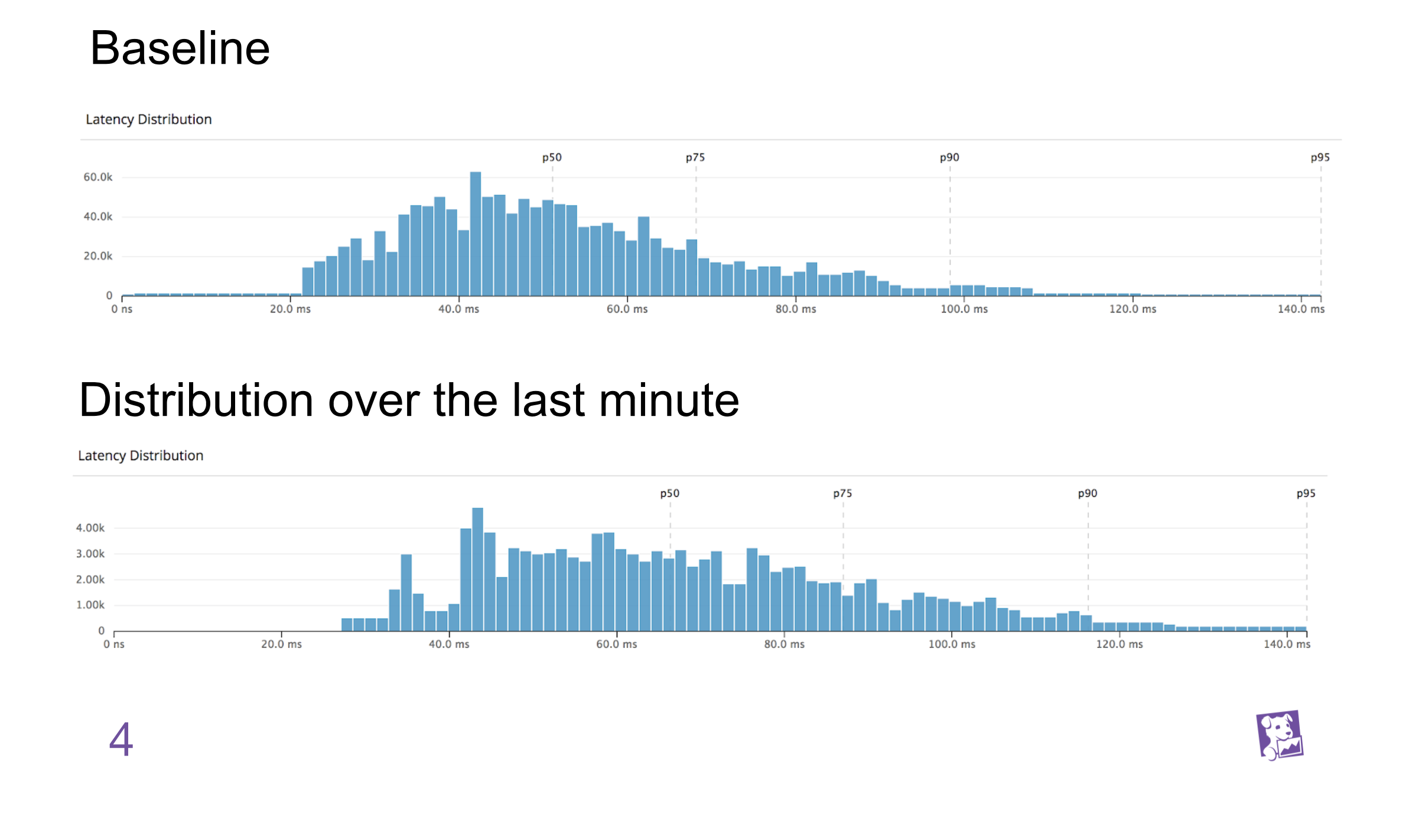## Probability distribution¶

• $K$: State space (For instance, $K=\mathbf{R}$ or compact subset in $\mathbf R^d$)
• $\mathcal F$: $\sigma$-algebra(field) on $K$

• $K\in \mathcal F$
• If $A\in \mathcal F$, then $A^c \in \mathcal F$
• closed under countable union
• Probability measure $\mathbf P$ on $(K, \mathcal F$)

• set function $\mathbf P : \mathcal F \to \mathbf R^+$ with $\mathbf P(K)=1$ (non negativity, null empty set, countable additivity)
• Probability distribution can be regarded as probability space $(K,\mathcal F,\mathbf P)$

## Example1(continuous)¶

• $K = \mathbf R^d$, $\mathcal F$ $=\mathcal B(\mathbf R^d)$, Borel sets
• For $A\in \mathcal F$,
$$\mathbf P(A):= \int_A p(x) dx$$

where

$$p(x)=\frac{1}{\sqrt{(2\pi)^d |\Sigma|}} \exp \left(-\frac{1}{2} (x-\mu)^T \Sigma^{-1}(x-\mu) \right),$$

mean vector $\mu\in \mathbf R^d$, covariance matrix $\Sigma \in \mathbf R^{d\times d}$, and det$(\Sigma)=|\Sigma |$.

## KS distance in SciPy¶

scipy.stats.ks_2samp(data1, data2)


Computes the Kolmogorov-Smirnov statistic on 2 samples.

This is a two-sided test for the null hypothesis that 2 independent samples are drawn from the same continuous distribution.

• Parameters
• data1, data2: sequence of 1-D ndarrays, two arrays of sample observations assumed to be drawn from a continuous distribution, sample sizes can be different
• Returns
• statistic : float, KS statistic
• pvalue : float, two-tailed p-value

## Example¶

from scipy import stats
n1 = 200
n2 = 300


For a different distribution, we can reject the null hypothesis since the pvalue is below 1%:

rvs1 = stats.norm.rvs(size=n1, loc=0., scale=1)
rvs2 = stats.norm.rvs(size=n2, loc=0.5, scale=1.5)
stats.ks_2samp(rvs1, rvs2)

(0.20833333333333337, 4.6674975515806989e-005)

## scipy.stats.ks_2samp(data1, data2)¶

data1 = np.sort(data1)
data2 = np.sort(data2)
n1 = data1.shape
n2 = data2.shape
data_all = np.concatenate([data1, data2])
cdf1 = np.searchsorted(data1, data_all, side='right') / (1.0 * n1)
cdf2 = np.searchsorted(data2, data_all, side='right') / (1.0 * n2)
d = np.max(np.absolute(cdf1 - cdf2))
# Note: d absolute not signed distance
en = np.sqrt(n1 * n2 / float(n1 + n2))
try:
prob = distributions.kstwobign.sf((en + 0.12 + 0.11 / en) * d)
except:
prob = 1.0

return Ks_2sampResult(d, prob)

In :
cum_plot()

KS distance 0.29533333333333334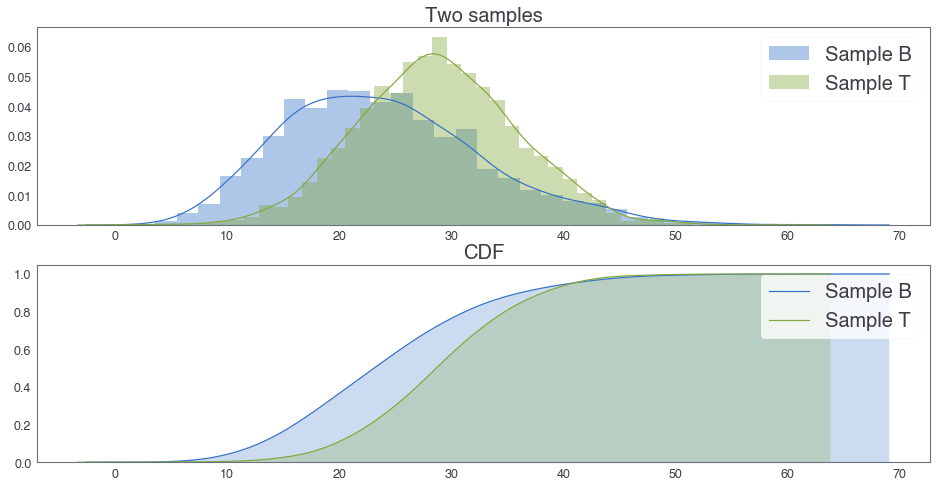In :
cum_plot(kde = False, bins = 1)

KS distance 0.5001666666666666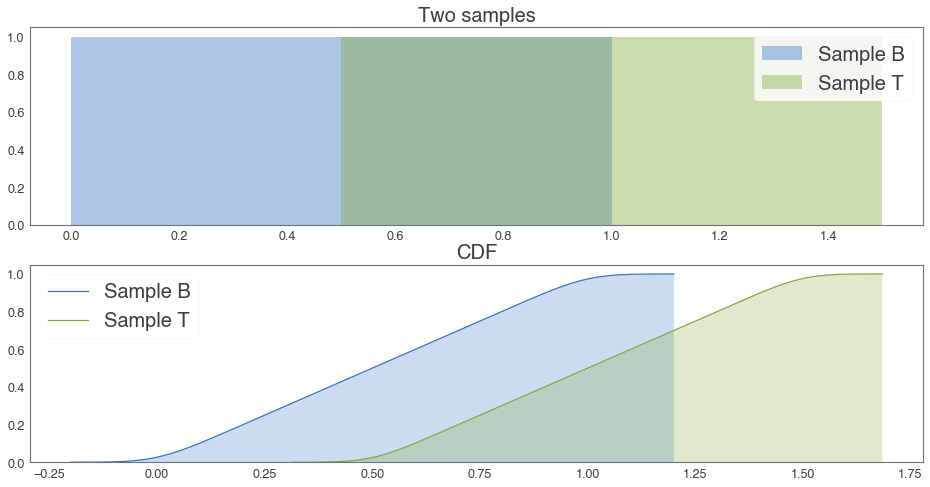## KS distance between two uniform distributions¶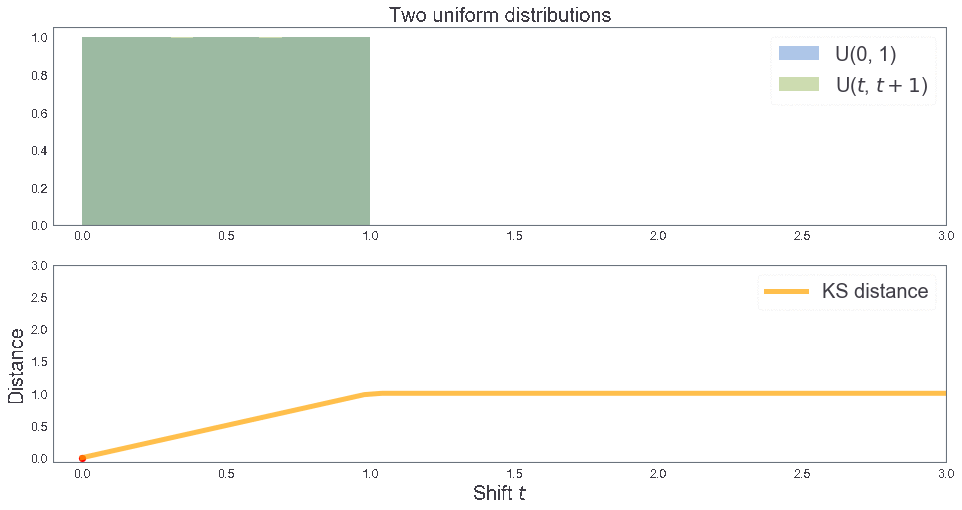## 3 samples: B, T, T'¶

In :
two_cdfs()

KS distance 0.1996667777407531
KS distance 0.1996667777407531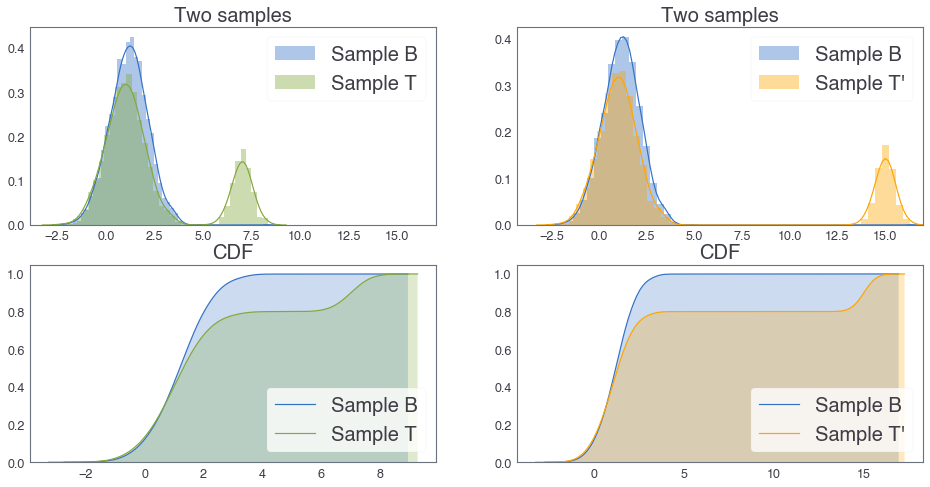## Need: more appropriate distance¶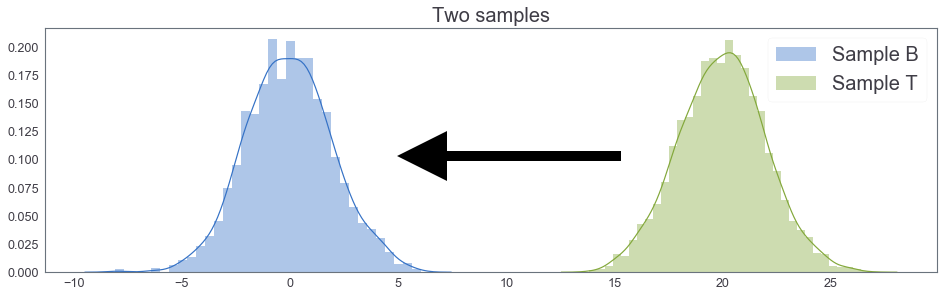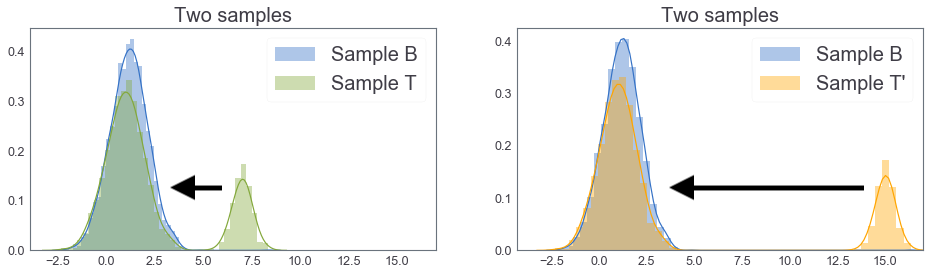## Joint Probability distribution with marginal $B$ and $T$¶

### Continuous version¶

$$\Gamma(B, T):= \left \{ \gamma: K \times K \longrightarrow \mathbf R^+\text{ s.t. }\int_K \gamma(x,y)dy = B(x)\text{ and }\int_K \gamma(x,y)dx = T(y) \right \}$$

where $B(x)$ (resp. $T(y)$) is a density function of $B$(resp. $T$).

$\Gamma(B,T)$ is a set of pdf on $K\times K$ whose marginal dfs are $B$ and $T$.

### Discrete version¶

$$\Gamma(B, T):= \left \{ \gamma: K \times K \longrightarrow [0,1]\text{ s.t. }\sum_{y\in K} \gamma(x,y) = B(x)\text{ and }\sum_{x \in K} \gamma(x,y) = T(y) \right \}$$

where $B(x)$ (resp. $T(y)$) is a probability mass function of $B$(resp. $T$).

$\Gamma(B,T)$ is a set of pmf on $K\times K$ whose marginal pmf are $B$ and $T$.

## Example¶

$K=\{1,2,3\}$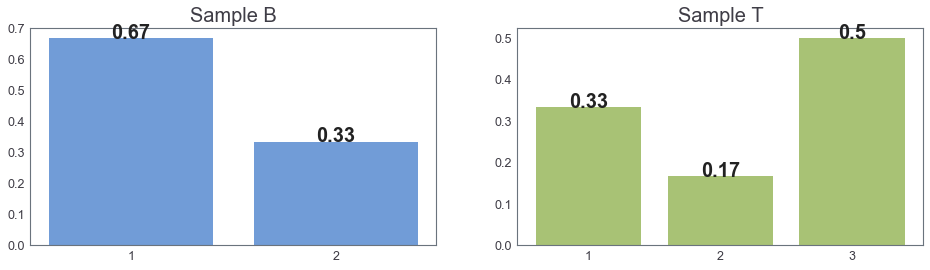## Earth mover's distance a.k.a. $1^{st}$ Wasserstein distance¶

(introduced by Leonid Vaseršteĭn(Russia))

\begin{align*} EMD(B,T) = W_1 (B,T) &= \inf_{\gamma \in \Gamma(B,T)} \int_{K\times K} |x-y| d\gamma(x,y) \quad \text{(continuous)}\\ & \\ &=\inf_{\gamma \in \Gamma(B,T)} \sum_{x,y} |x-y| \gamma(x,y) \quad \text{(discrete)} \end{align*}

#### Remark¶

• $EMD(B,T) = \inf_{\gamma \in \Gamma(B,T)} E_{(x,y) \sim \gamma} \left [ |x-y| \right ]$
• $\gamma(x, y)$ can be seen as an amount of sand to move from $x \in B$ to $y \in T$
• Intuitively, $EMD$ is a minimum cost to move sand to another region(Optimal transport problem)

## $p^{th}$ Wasserstein distance¶

For $p\geq 1$, $p^{th}$ Wasserstein distance is defined as

$$W_p (B,T) = \inf_{\gamma \in \Gamma(B,T)} \left (\int_{K\times K} |x-y|^p d\gamma(x,y) \right)^{\frac{1}{p}}$$

### Remark¶

• $p^{th}$ Wasserstein distance is a metric.

## Another formulation $\color{red}{only}$ in case $K = \mathbf R$¶

Let $K = \mathbf R$. Then we have another formulation:

$$EMD(B,T) = \int_{\mathbf R} |F_B(x) - F_T(x)| dx$$

where $F_B$ is the CDF of $B$.

• Ref: On Wasserstein Two Sample Testing and Related Families of Nonparametric Tests

For your information, KS distance was the following:

$$KS(B, T):= \sup_{x\in \mathbf R} \left | F_B(x) - F_T(x) \right|$$

### Remark¶

• $B$$= \delta_s and T$$=$ $\delta_t$, $s,t$ $\in$ $\mathbf R$
$$\delta_s: \text{ Dirac delta distribution at }s.$$

#### $\quad \Rightarrow EMD(B, T)=|s-t|.$¶

• $B=N(\mu_1, C_1)$ and $T=N(\mu_2, C_2)$ on $\mathbf R^d$ where mean vectors $\mu_1, \mu_2 \in \mathbf R^d$, covariance matrices $C_1, C_2 \in \mathbf R^{d\times d}$.

## 1st Wasserstein distance in SciPy¶

scipy.stats.wasserstein_distance(u_values, v_values, u_weights=None, v_weights=None)


Compute the first Wasserstein distance between two 1D distributions.

• Parameters
• u_values, v_values: array_like, Values observed in the (empirical) distribution.
• u_weights, v_weights: array_like, optional, Weight for each value. If unspecified, each value is assigned the same weight. u_weights (resp. v_weights) must have the same length as u_values (resp. v_values). If the weight sum differs from 1, it must still be positive and finite so that the weights can be normalized to sum to 1.
• Returns
• distance: float, The computed distance between the distributions.
In :
two_cdfs2()

EMD between B and T: 1.0263053460000136
EMD between B and T': 2.623639567926038## EMD between two uniform distributions¶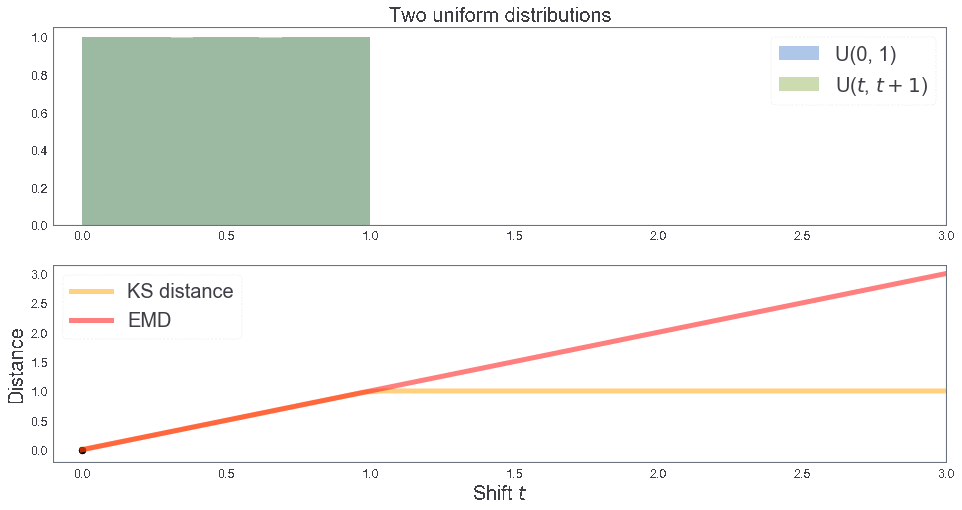In :
B = [1, 1, 2] # Sample B
T = [1, 1, 2, 3, 3, 3] # Sample T

print("EMD between B and T:", stats.wasserstein_distance(B,T))

v1, w1 = [1, 2], [2, 1] # Sample B
v2, w2 = [1, 2, 3], [2, 1, 3] # Sample T

print("EMD between B and T:", stats.wasserstein_distance(v1, v2, w1, w2))

EMD between B and T: 0.8333333333333333
EMD between B and T: 0.8333333333333333


## 2018년 국립중앙과학관 자연사관의 시간별 방문객 수 샘플¶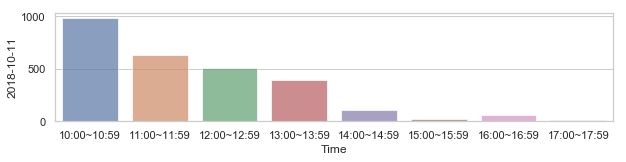## 5월 5일과 나머지 날짜의 방문객 수 분포 차이 측정¶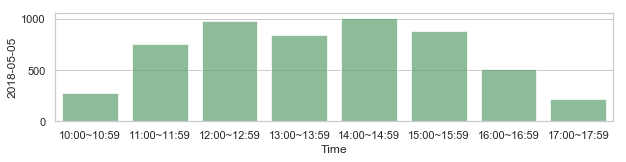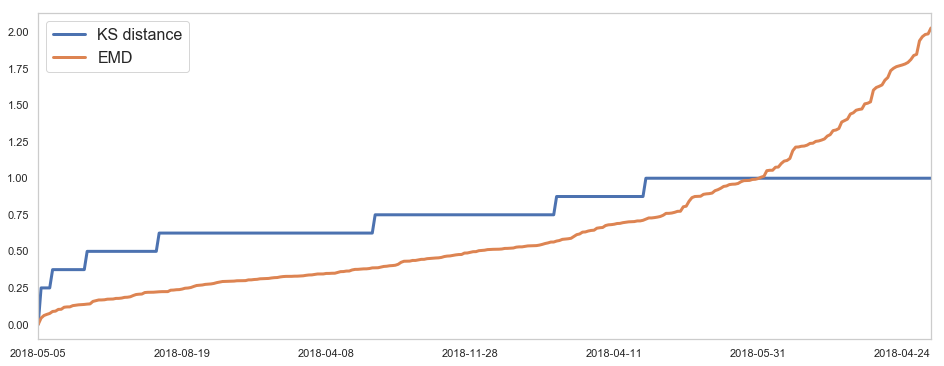## 5월 5일 방문객 수 분포와의 유사도 측정(KS-distance)¶

### 짧은 거리 순¶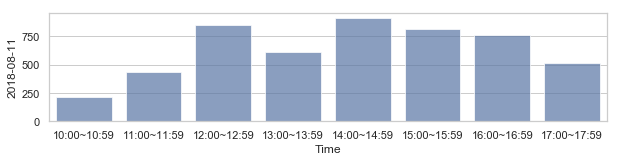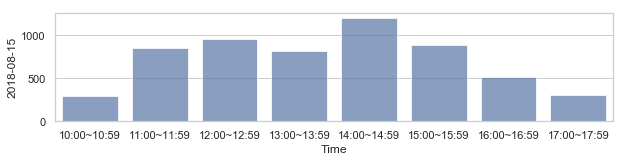## 5월 5일 방문객 수 분포와의 유사도 측정(KS-distance)¶

### 먼 거리 순¶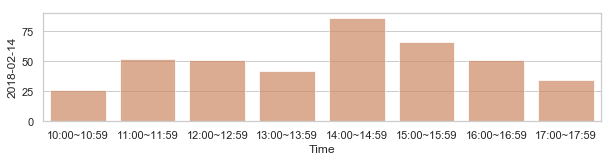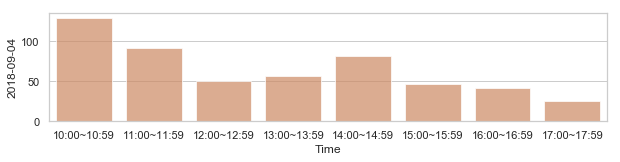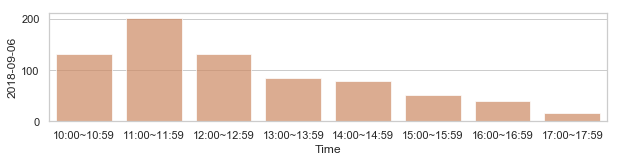## 5월 5일 방문객 수 분포와의 유사도 측정(EMD)¶

### 짧은 거리 순¶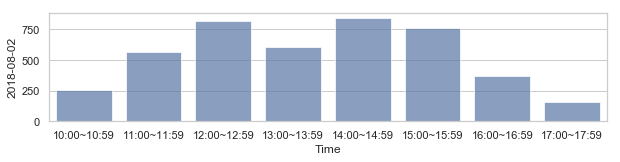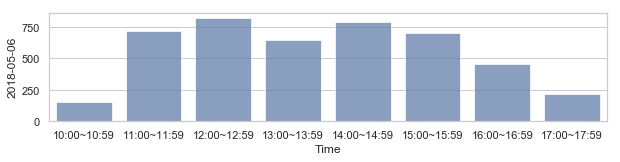## 5월 5일 방문객 수 분포와의 유사도 측정(EMD)¶

### 먼 거리 순¶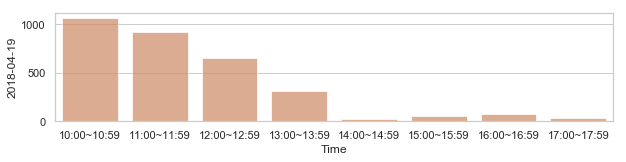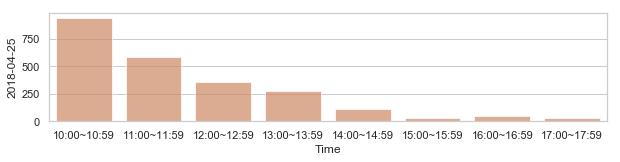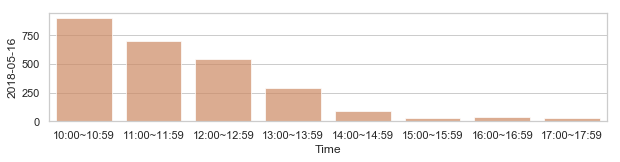## 부산지역 582,184 세대별 도시가스 사용량¶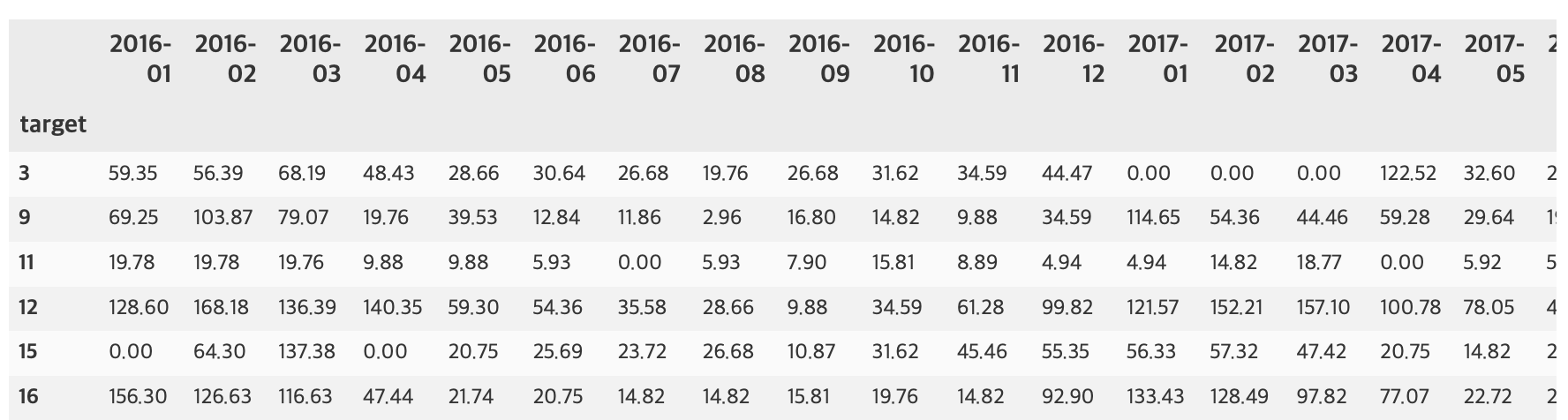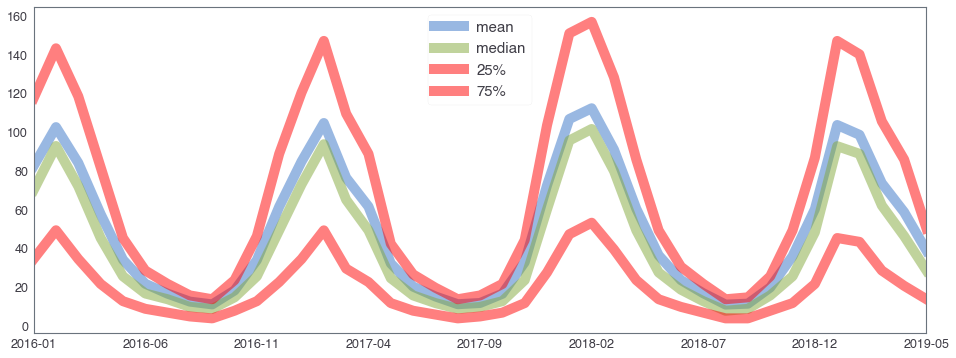## 582,184 세대 평균과 유사한 패턴을 띄는 세대 top 50¶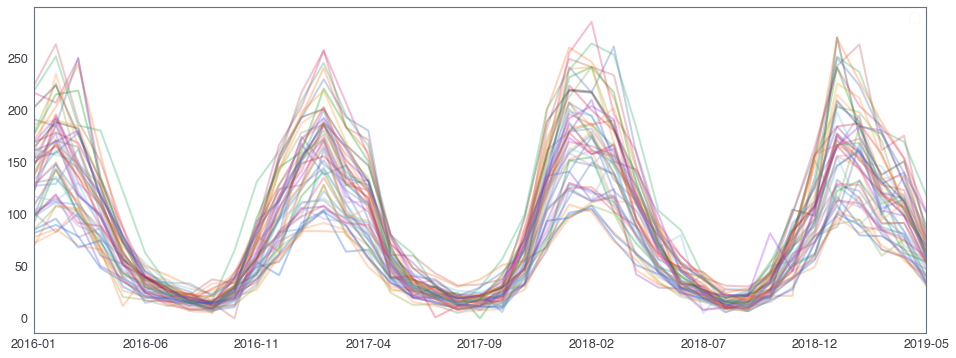## 582,184 세대 평균과 다른 패턴을 띄는 세대 top 2000¶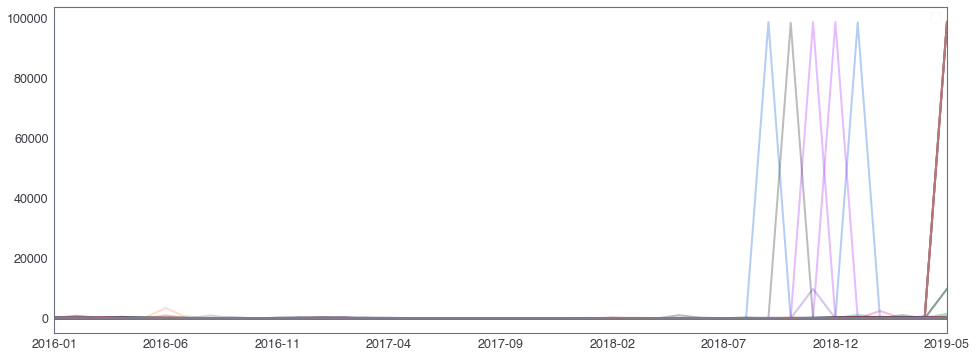## 58,2184 세대 평균과 다른 패턴을 띄는 세대¶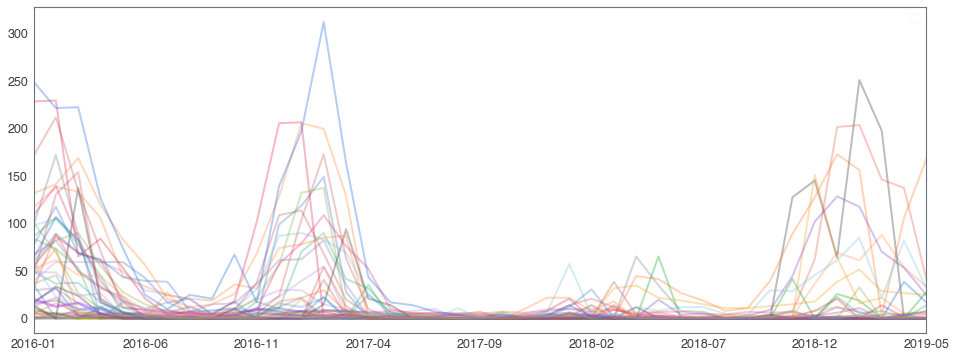## Earth mover's distance with Linear programming¶

Treat each sample set $B$ corresponding to a “point” as a discrete probability distribution, so that each sample $x \in B$ has probability mass $p_x = 1 / |B|$. The distance between $B$ and $T$ is the optional solution to the following linear program.

Each $x \in B$ corresponds to a pile of dirt of height $p_x$, and each $y \in T$ corresponds to a hole of depth $p_y$. The cost of moving a unit of dirt from $x$ to $y$ is the Euclidean distance $d(x, y)$ between the points (or whatever hipster metric you want to use).

Let $\gamma(x, y)$ be a real variable corresponding to an amount of dirt to move from $x \in B$ to $y \in T$, with cost $d(x, y)$. Then the constraints are:

• Each $\gamma(x, y) \geq 0$, so dirt only moves from $x$ to $y$.
• Every pile $x \in B$ must vanish, i.e. for each fixed $x \in B$, $\sum_{y \in T} \gamma(x, y) = p_x$.
• Likewise, every hole $y \in T$ must be completely filled, i.e. $\sum_{x \in B} \gamma(x, y) = p_y$.

The objective is to minimize the cost of doing this: $\sum_{x, y \in B \times T} d(x, y) \gamma(x, y)$.

import math
import numpy as np
from collections import Counter
from collections import defaultdict
from ortools.linear_solver import pywraplp

def euclidean_distance_2(x, y):
return math.sqrt(sum((a - b) ** 2 for (a, b) in zip(x, y)))

def euclidean_distance_1(x, y):
return math.sqrt((x - y) ** 2 )

def earthmover_distance(p1, p2):

if np.array(p1).shape[-1] == 2:
euclidean_distance = euclidean_distance_2
else:
euclidean_distance = euclidean_distance_1
'''
Output the Earthmover distance between the two given points.
'''
dist1 = {x: count / len(p1) for (x, count) in Counter(p1).items()}
dist2 = {x: count / len(p2) for (x, count) in Counter(p2).items()}

if len(set(p2).difference(p1)) >0:
for x in set(p2).difference(p1):
dist1[x] = 0.

if len(set(p1).difference(p2)) >0:
for x in set(p1).difference(p2):
dist2[x] = 0.

solver = pywraplp.Solver("earthmover_distance",
pywraplp.Solver.GLOP_LINEAR_PROGRAMMING)

variables = dict()

dirt_leaving_constraints = defaultdict(lambda: 0)
dirt_filling_constraints = defaultdict(lambda: 0)

# the objective
objective = solver.Objective()
objective.SetMinimization()

for (x, dirt_at_x) in dist1.items():
for (y, capacity_of_y) in dist2.items():
amount_to_move_x_y = solver.NumVar(0,
solver.infinity(), "z_{%s, %s}" % (x, y))
variables[(x, y)] = amount_to_move_x_y
dirt_leaving_constraints[x] += amount_to_move_x_y
dirt_filling_constraints[y] += amount_to_move_x_y
objective.SetCoefficient(amount_to_move_x_y,
euclidean_distance(x, y))

for x, linear_combination in dirt_leaving_constraints.items():

for y, linear_combination in dirt_filling_constraints.items():

status = solver.Solve()
if status not in [solver.OPTIMAL, solver.FEASIBLE]:
raise Exception("Unable to find feasible solution")

for ((x, y), variable) in variables.items():
if variable.solution_value() != 0:
cost = euclidean_distance(x, y) * variable.solution_value()

return objective.Value()

In :
v1 = [1, 1, 2]

v2 = [1, 1, 2, 3, 3, 3]

print(earthmover_distance(v1, v2))
print(stats.wasserstein_distance(v1, v2))

0.8333333333333334
0.8333333333333333

In [ ]:
print(earthmover_distance(rvs3[: : 3], rvs4[: : 3]))
# >>> 29 s ± 139 ms per loop (mean ± std. dev. of 7 runs, 1 loop each)

print(stats.wasserstein_distance(rvs3[: : 3], rvs4[: : 3]))
# >>> 424 µs ± 2.81 µs per loop (mean ± std. dev. of 7 runs, 1000 loops each)


## The learning a probability distribution of a data is very important for machine learning¶

• $\mathbf P_r$: target prob. measure(distribution)
• $\mathbf P_\theta$: prob. measure parametrized by $\theta$

### Maximum Likelihood method¶

$$\max \color{red}{\text{Likelihood}} \iff \min \color{red}{KL(\mathbf P_r | \mathbf P_\theta)} \iff \min\color{red}{\text{cross-entropy}}$$

However, $KL$-divergence sometimes overshoot!

## Relation between distances¶

### Statistical distances¶

• total variation distance, $\delta(\mathbf P, \mathbf P_n)$
• KL-divergence, $KL(\mathbf P | \mathbf P_n)$
• Wasserstein distance, $W(\mathbf P, \mathbf P_n)$

### Relation¶

• $\mathbf P$: fixed prob. measure on $(K, \mathcal F)$
• $(\mathbf P_n)_n$: seq. of prob. distributions on $(K, \mathcal F)$

Let $n\to \infty$, then $$\delta(\mathbf P, \mathbf P_n) \to 0 \iff KL(\mathbf P | \mathbf P_n) \to 0 \iff KL(\mathbf P_n | \mathbf P) \to 0. \qquad (*)$$

Furthermore, $(*)$ with $n\to \infty$ implies

$$W(\mathbf P, \mathbf P_n) \to 0.$$

## Finite signed measure space¶

Let $K\subset \mathbf R^d$ be a compact and $\mathcal F:= \mathcal B(K)$. Let $\mu$ be a finite signed measure on $(K, \mathcal F)$ (by Hahn decomposition, uniquely devided by finite measures $\mu_+, \mu_-$) and total variation norm

\begin{align*} \| \mu \|_{TV}:&= \sup_{A\in \mathcal F} |\mu(A)| + |\mu(A^c)|\\ &= \mu_+(K) + \mu_-(K) \end{align*}

Let $$\mathcal M := \left \{ \mu :\text{finite signed measure on }(K, \mathcal F) \right \}.$$

#### Then $\mathcal M$ is a Banach space w.r.t. $\| \mu \|_{TV}$.¶

$$Prob(K):=\text{ all prob. measures on }(K, \mathcal F).$$

Then $Prob(K)$ is a closed subspace of $\mathcal M$, complete itself.

For $\mu, \nu \in Prob(K)$, total variation distance defined by

$$\delta(\mu, \nu):= \| \mu - \nu \|_{TV}= \sup_{A\in \mathcal F} |\mu(A) - \nu(A)| + |\mu(A^c)-\nu(A^c)|$$

is a distance in $Prob(K)$.

## Continuous function space on a compact set $K$ and its dual¶

Consider

$$C(K):= \{ f: K \to \mathbf R, \text{ continuous} \}$$

with norm $\| f\|_\infty := \max_{x\in K} |f(x)|$. Then $(C(K), \| \cdot \|_\infty)$ is a normed space. Define its dual

$$C(K)^*:= \{ \phi: C(K) \to \mathbf R, \text{ linear and continuous} \}$$

with dual norm $\| \phi \| := \sup_{f\in C(K), \, \|f \|_\infty\leq 1} |\phi(f)|$.

## Isometric immersion¶

$$\Phi: (Prob(K), \delta) \longrightarrow (C(K)^* , \| \cdot \|)$$$$\Phi(\mu)(f) = \int_K f d\mu, \quad\text{ for }\, f \in C(K), \, \mu\in Prob(K)$$

Then $\Phi$ is an isometric immersion.

##### It means that $(Prob(K), \delta)$ can be as a subset of $(C(K)^*, \|\cdot \|)$ or total variation distance $\delta$ over $Prob(K)$ is exactly the norm distance over $C(K)^*$.¶

Dual space $C(K)^*$ has a weak$^*$ topology which is weaker than the strong topology induced by $\| \cdot \|$.

##### In the case of $Prob(K) (\subset C(K)^*)$, the strong topology is given by the total variation, but weak$^*$ topology is given by the Wasserstein distance.¶

Consider two probability measure $\mathbf P, \mathbf Q$ on $(K, \mathcal F)$.

#### 1. Total variation distance¶

$$\delta(\mathbf P, \mathbf Q):= \| \mathbf P - \mathbf Q \|_{TV} = \sup_{A\in \mathcal F} | \mathbf P(A) - \mathbf Q(A)| + |\mathbf P(A^c)-\mathbf Q(A^c)|$$

#### 2. $KL$-divergence(Kull back-Leibler)¶

$$KL(\mathbf P | \mathbf Q) := \int_K \log \left (\frac{p(x)}{q(x)} \right) p(x) \mu (dx)$$

where $\mathbf P$ and $\mathbf Q$ are absolutely continuous w.r.t. $\mu$ on $K$ $(A\in \mathcal F$ if $\mu(A) = 0$, then $\mathbf P(A) = \mathbf Q(A) = 0)$.

#### 3. Jensen-Shannon(JS) divergence¶

$$JS(\mathbf P, \mathbf Q):= KL(\mathbf P | \mathbf M) + KL(\mathbf Q | \mathbf M)$$

where $\mathbf M:= (\mathbf P + \mathbf Q)/2$.

#### 4. EM distance, 1$^{st}$ Wasserstein distance¶

$$\inf_{\gamma \in \Gamma(\mathbf P, \mathbf Q)} E_{(x,y)\sim \gamma} [|x-y|]$$

### Let $\mathbf P$ be a prob. measure on $K$ and $(\mathbf P_n)_n$ be a seq. of prob. measures on $K$.¶

#### 1. The following statements are equivalent.¶

\begin{align*} & \delta(\mathbf P_n , \mathbf P) \to 0 \text{ as }n \to \infty.\\ & \qquad \qquad \qquad\qquad\qquad\qquad (*)\\ & JS(\mathbf P_n , \mathbf P) \to 0 \text{ as } n \to \infty. \end{align*}

#### 2. The following statements are equivalent.¶

\begin{align*} & W(\mathbf P_n , \mathbf P) \to 0 \text{ as }n \to \infty.\\ & \\ & \mathbf P_n \to \mathbf P\text{ converges in distribution for r.v. as }n \to \infty \end{align*}

## Reference¶

• On Wasserstein Two-Sample Testing and Related Families of Nonparametric Tests
• Concrete representation of abstract (m)-spaces (a characterization of the space of continuous functions)
• Optimal Transport
• Wasserstein GAN
• Earthmover Distance: https://jeremykun.com/tag/wasserstein-metric/